Often times when you work with excel you can use these hacks (simple formulas) in your larger formulas to ease your work. I could think of 25 of them but I am sure there are many. Why don’t you go through these and add to list the ones that you use most often..

1. Count Blank Cells in a Range using COUNTBLANKUse this formula for counting only blank cells in a range

2. Count Text in a Range using COUNTA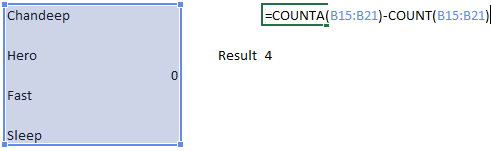Note that COUNTA will count everything (text and numbers) and COUNT will only count numbers. The difference of these two will give you the number of text items in a range

3. Use Wild cards with SUMIF/COUNTIF for approximation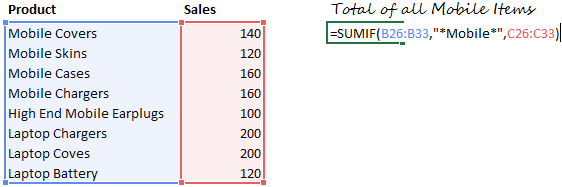Notice the asterisk (*) sign before and after the word “Mobile” that means there could be anything written before or after the word Mobile. Similar logic can be applied in COUNTIF as well

Related : Learn Countif and Sumif

4. Sum by Clubbing worksheets in a FormulaThis is will take a total from Sheet 4 to Sheet 2 of the cell A1 on each sheet

5. Rounding off to the next multiple of 100 using CEILING FunctionRelated : Learn Ceiling Function

6. Rounding off to the previous multiple of 50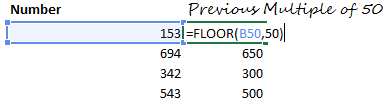Related : Learn Floor Function

7. Stripping off decimals from a Number using INT FunctionRelated : Learn Int Function

8. Keeping only Decimals using MOD and INTRelated : Learn Int and Mod Function

9. Getting the name of the month from a Date using TEXT10. Converting values into other11. Generating Random NumbersRelated : Learn Randbetween Function

12. Generating Random Alphabets (Caps and Non Caps)1. Note that numbers 65 to 90 will return capital letters
2. For lower case letters use the numbers 97 (for a) and 122 (for z) in the same formula

13. Retrieving the File Name Using the CELL, MID and SEARCH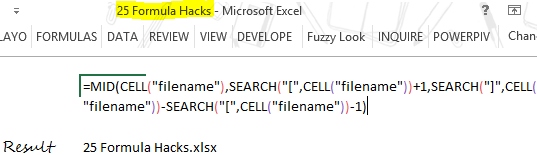14. Calculating EMIs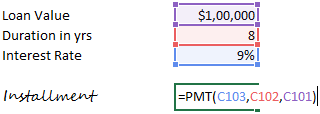15. Matching data EXACTLYNote that the EXACT Function not only matches the values but even the case (upper or lower) of the text. If the 2 values do not match, it returns a FALSE

16. Removing Extra Spaces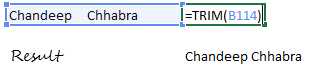The Trim Function leaves one space by default between 2 words. Read this when your TRIM function fails to remove spaces

17. Changing the case of the TEXT – UPPER LOWER or PROPER18. Get the Column or Row number of any cellIf you do not write anything in the ROW or COLUMN function, it will return the row or the columns number of the cell in which the formula is written

Related : Learn Row and Column function

19. Transpose your data – Horizontal to vertical or vice versa1. First Select the range where you want to write the transpose the date
2. Then write the Transpose formula
3. The Press CTRL SHIFT and ENTER to execute the formula

Related : Learn Transpose FunctionA hyperlink named Blog will be generated will which lead you to www.goodly.co.in

21. Extracting Formulas from the Cells22. Finding the Value of PI23. Find the power of any numberThis means 2 to the power of 5. Which can also be written as =2^5

24. Finding the Sq Root of any number25. Finding the QuotientRelated : Learn

1. Quotient Function
2. Use quotient for finding Quarter and Indian Financial year Quarter

The Last one is a Bonus and you’ll love it!

26. Converting a number of Roman NumbersCan you guess the result.. sure enough it is XL

## Other Formula Resources

What your favorite formula hacks in Excel?